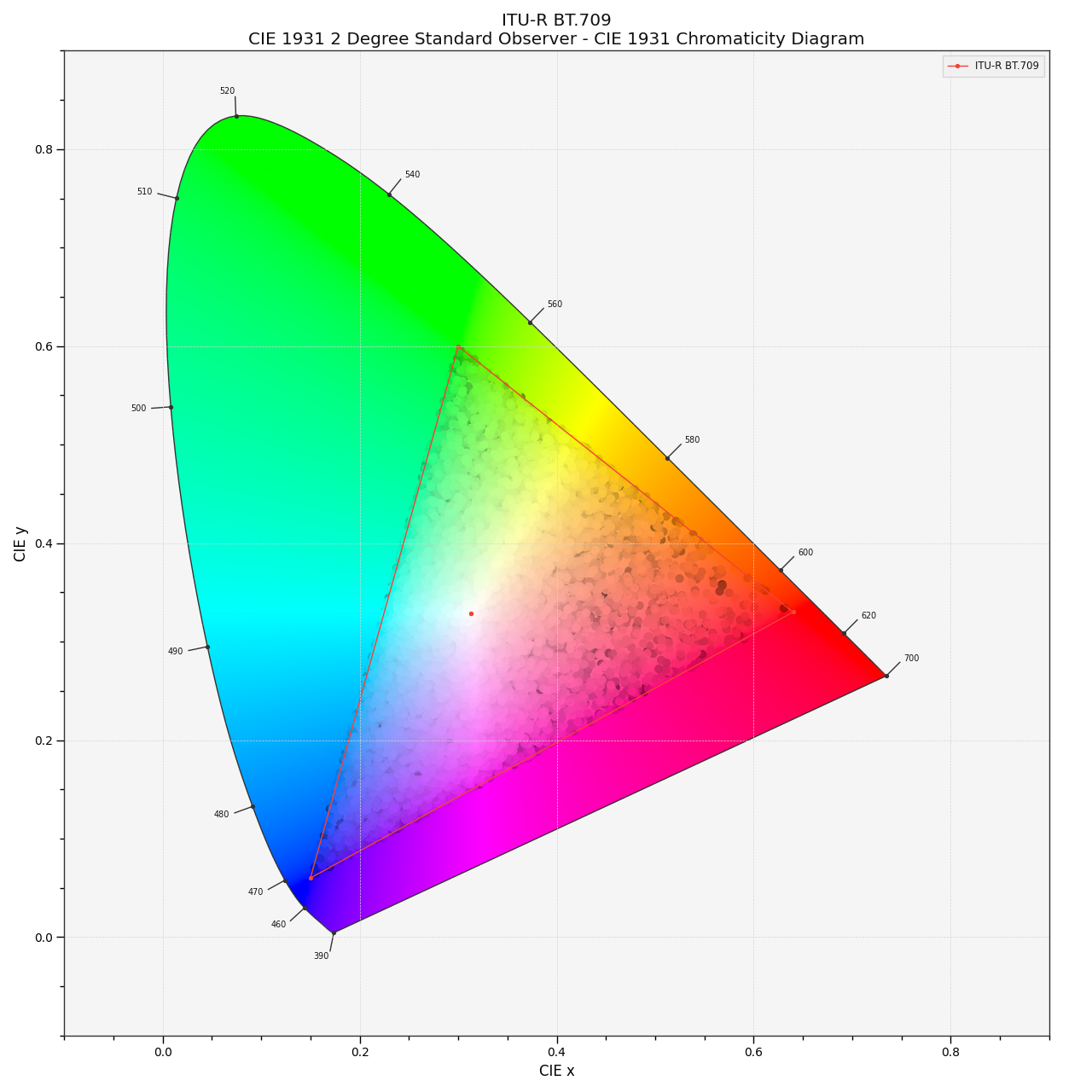# colour.plotting.models.plot_RGB_chromaticities_in_chromaticity_diagram#

colour.plotting.models.plot_RGB_chromaticities_in_chromaticity_diagram(RGB: ArrayLike, colourspace: = 'sRGB', chromaticity_diagram_callable: Callable = plot_RGB_colourspaces_in_chromaticity_diagram, method: Literal['CIE 1931', 'CIE 1960 UCS', 'CIE 1976 UCS'] | str = 'CIE 1931', scatter_kwargs: = None, **kwargs: Any) [source]#

Plot given RGB colourspace array in the Chromaticity Diagram according to given method.

Parameters:
Returns:

Current figure and axes.

Return type:

`tuple`

Examples

```>>> RGB = np.random.random((128, 128, 3))
>>> plot_RGB_chromaticities_in_chromaticity_diagram(RGB, "ITU-R BT.709")
...
(<Figure size ... with 1 Axes>, <...Axes...>)
```